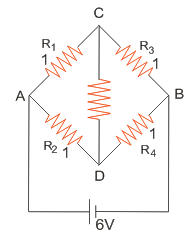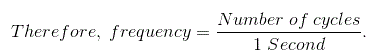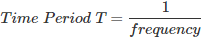# MCQs on Electric Circuits

##### Page 13 of 63. Go to page 1 2 3 4 5 6 7 8 9 10 11 12 13 14 15 16 17 18 19 20 21 22 23 24 25 26 27 28 29 30 31 32 33 34 35 36 37 38 39 40 41 42 43 44 45 46 47 48 49 50 51 52 53 54 55 56 57 58 59 60 61 62 63
01․ Which property is used to oppose the flow of current ?
Poteintial difference
Capacitance
Inductance
Resistance

Resistance is the property of a element which opposes of flow of electric current only the magnitude, whereas inductance opposes the flow of both the direction and magnitude of current.

02․ What is the value of charge of an electron?
1.6022 × 10-19 Coulomb
1.6022 × 10-27 Coulomb
1.6022 × 10-8 Coulomb
1.6022 × 10-7 Coulomb

Charge of an electron is 1.6022 × 10-19 Coulomb.

03․ What is the SI unit of electric current ?
Ampere.
Ohm.
None of the above.

SI unit of electric current is Ampere. A current of one ampere is defined as one coulomb of charge going through a given point per second. It is named after a French mathematician Andre Ampere.

04․ There is a ________ between two nodes of signal flow graph
branch
tree
none of the above.

A node can be defined as a connection point between two or more branches. Hence, there should be a branch in between the two nodes of signal flow graph.

05․ The current through the 2 KΩ resistance ( rest resitances are all 1 ohm each ) in the circuit shown in diagram0 mA.
1 mA.
2 mA.
6 mA.

Bridge is balanced i.e node C and D are at same potential. No current flows through 2 KΩ resistor. Balanced bridge can be obtained when R1R4 = R2R3.

06․ What is the dielectric material used in precision small value capacitor and capacitor used in radio frequency circuits?
Oil impregnated paper.
Glass.
Vacuum, air.
Titanium oxide.

Except the vacuum and air, the above all dielectric materials are used in high value capacitors. But, the precision small value capacitor and the capacitor used in radio frequency circuits are required less value of capacitor. So, in those capacitors, vacuum and air are used as a dielectric medium.

07․ In a series resonant circuit impedance is
minimum.
maximum.
zero.
none of these.

At series resonance condition, the inductive reactance balances the capacitive reactance, that means XL = XC. So, now impedance becomes equal to resistance only. In this condition impedance becomes minimum.

08․ What is time period T?
Time taken by an alternating quantity to complete the (+)ve half.
Time taken by an alternating quantity to complete one full cycle.
Time taken by an alternating quantity to complete the (-)ve half.
Time taken by an alternating quantity to reach the peak value.

We know that the magnitude of the alternating quantity varies continuously from the starting point of the positive cycle to the ending point of the negative cycle. So, the required time to complete one full cycle is called as time period T. In one time period AC completes one positive peak and one negative peak.

09․ What is called the frequency in AC operation?
No. of cycles made per second.
Basically, the frequency is nothing but the no. of completed cycles per unit time. A cycles contains one positive half cycles and one negative half cycles.Frequency is the completed cycles per second. So, the time period is inversely proportional to the frequency.